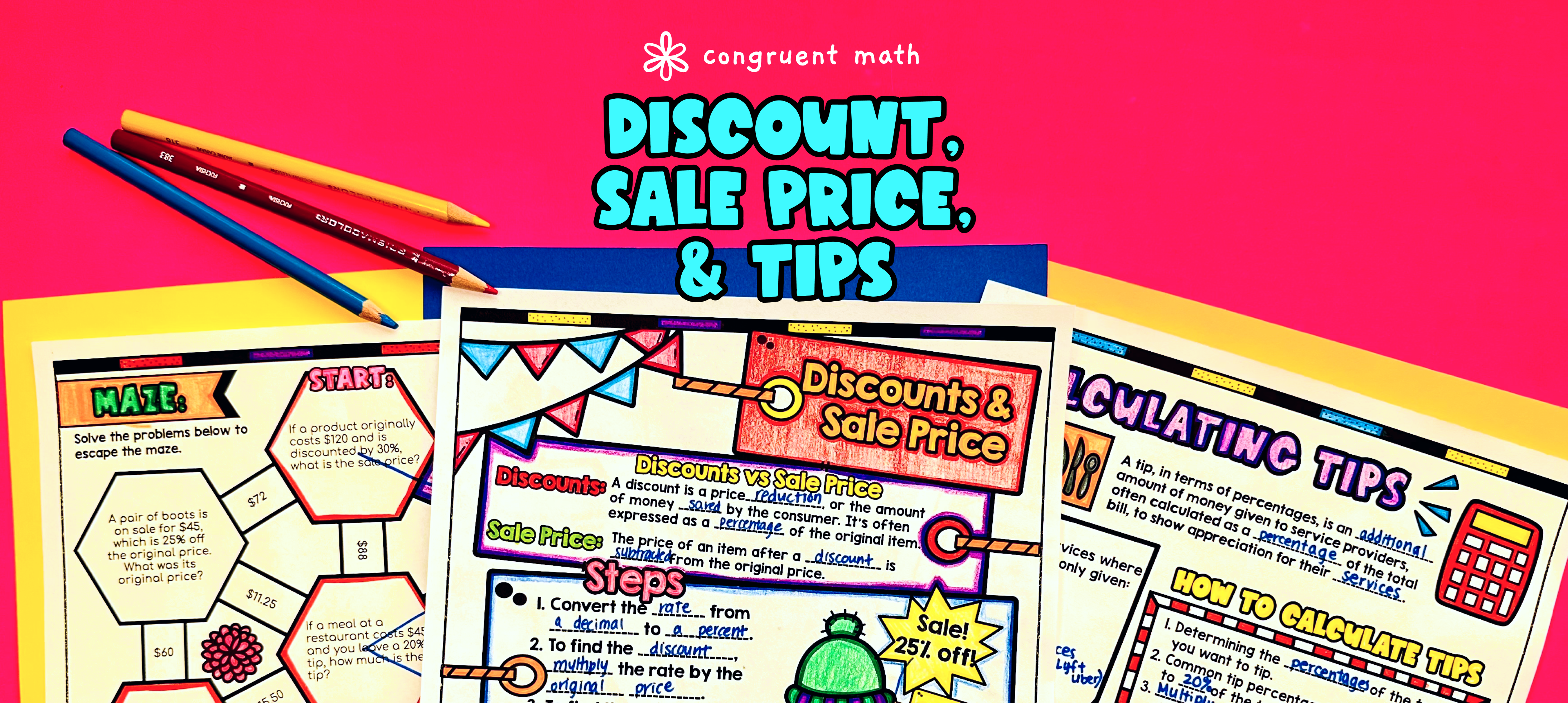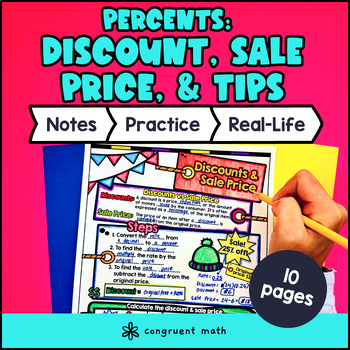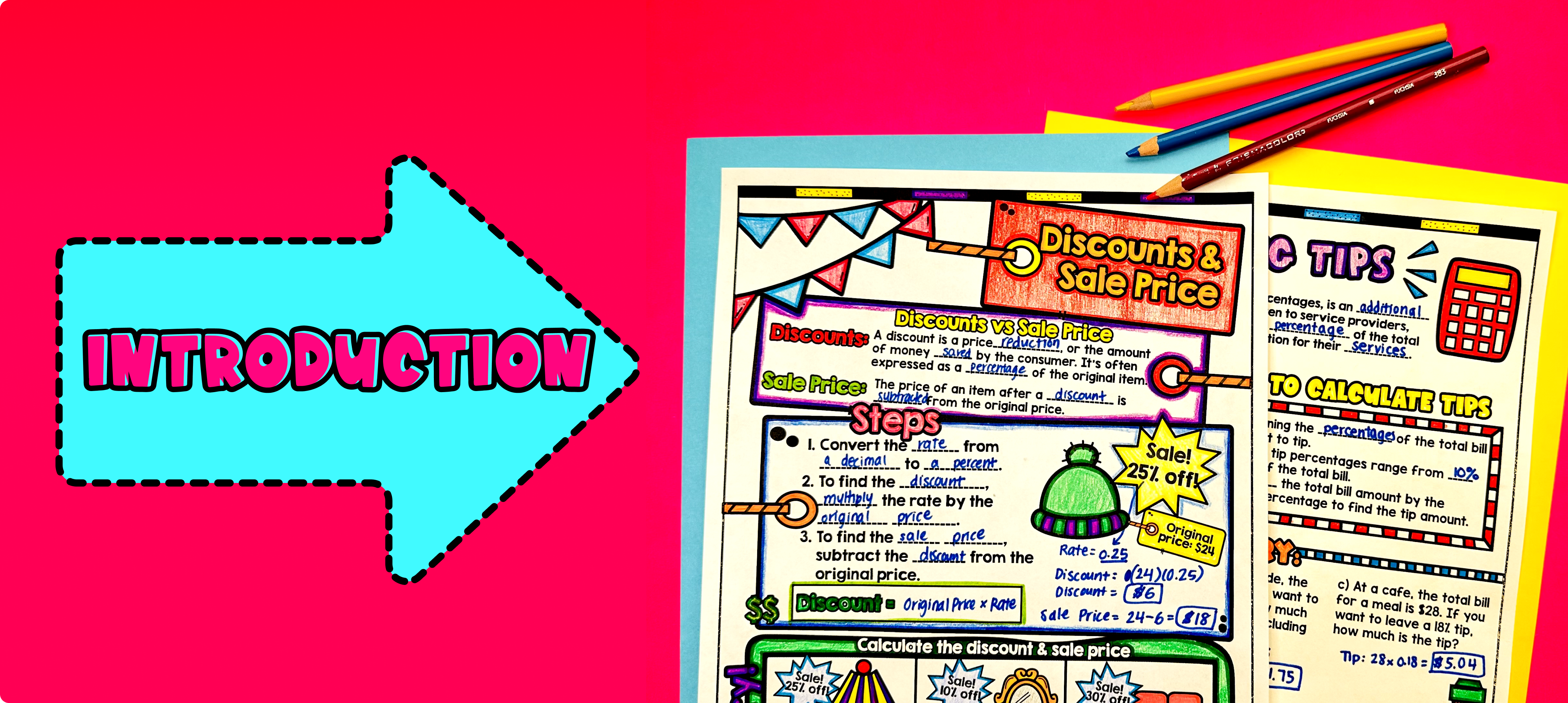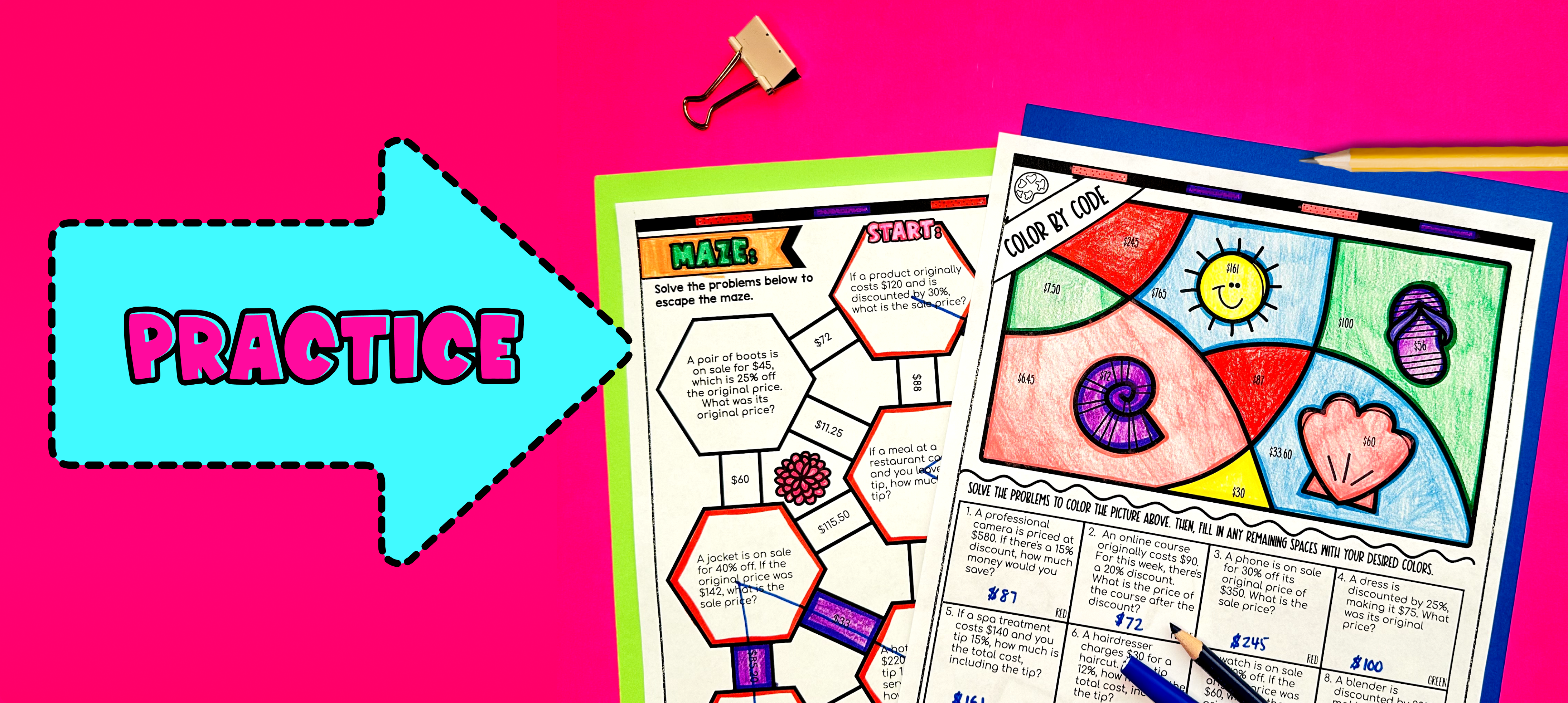# Percents Applications Discount, Sale Price, and Tips Lesson PlanPin This

## Overview

Ever wondered how to teach percent applications involving discount, sale price, and tips in an engaging way to your seventh grade students?

In this lesson plan, students will learn about calculating discount, finding sale prices, and determining tips, all using percentages. Through artistic and interactive guided notes, check for understanding questions, a doodle and color by number activity, and a maze worksheet, students will gain a comprehensive understanding of percent applications.

The lesson culminates with a real-life example that explores how calculating discounts and finding sale prices is useful in everyday situations, such as shopping or dining out. This real-life application will help students see the relevance and importance of understanding and using percentages in their daily lives.

## Get the Lesson Materials\$4.25

## Learning Objectives

After this lesson, students will be able to:

• Calculate the discount amount and sale price of an item given the original price and the percent discount.

• Solve problems involving finding the original price of an item given the sale price and the percent discount.

• Determine the total cost including tips based on a percentage of the bill amount.

• Apply the concepts of discount, sale price, and tips to real-life situations, such as shopping and dining out.

## Prerequisites

Before this lesson, students should be familiar with:

• How to convert between decimals and percents

• Basic understanding of multiplication and division of integers and decimals

• Discount

• Sale price

• Tips

• Percentage

## Procedure

### IntroductionPin This

As a hook, ask students why it is important to calculate discounts, sale prices, and tips in real life situations. Refer to the last page of the guided notes as well as the FAQs below for ideas.

Use the first page of the guided notes to introduce the concept of discounts. Walk through how to calculate the discount amount and the sale price. Explain the difference between the discount and the sale price. Have students fill in the steps to calculating discount and sale price. Then, have students practice using the 3 problems in the "you try" section on the page. Students will have to calculate the sale prices of the different furniture shown.

Next, use the second page of the guided notes to introduce tip calculations. Discuss the purpose of tipping and how it is typically calculated as a percentage of the total bill. Show students how to calculate the tip amount and the total amount, including the tip. Emphasize the importance of good tipping etiquette and have students fill in some services like waitressing, salons, and food delivery that often accept tips.

Based on student responses, reteach concepts that students need extra help with. If your class has a wide range of proficiency levels, you can pull out students for reteaching, and have more advanced students begin work on the practice exercises.

### PracticePin This

After finishing the first two pages of the guided notes, have students practice finding discounts, sale prices, and tips using the maze activity (page 3). Walk around the classroom to answer any questions students may have.

Fast finishers can dive into the color by number activity (page 4) for extra practice. You can assign it as homework for the remainder of the class.

### Real-Life ApplicationPin This

Bring the class back together, and introduce the concept of calculating tips and sale price in real-life situations. Explain that understanding how to find the tip when dining at a restaurant or calculating the sale price when shopping can be useful in everyday life. Use the last page of the guided notes where students will read about a detailed example of real life applications.

Provide examples and scenarios where calculating tips and sale prices are relevant. For example, you can discuss scenarios such as:

1. Dining at a restaurant: Explain that when dining out, it is customary to leave a tip for the server. Show the students how to calculate a specific percentage tip based on the total bill. Discuss common tipping percentages, such as 15%, 18%, and 20%, and emphasize the importance of considering the quality of service when determining the appropriate tip.

2. Shopping during a sale: Explain that when there is a sale at a store, the price of an item is often reduced by a certain percentage. Show the students how to calculate the sale price of an item by applying the discount percentage to the original price. Discuss the concept of finding the better deal between items with different sale prices and comparing the savings.

3. Discounts and coupons: Explain that discounts and coupons are often used to reduce the price of items. Show the students how to calculate the discounted price using a specific percentage discount or a fixed amount coupon. Discuss the importance of reading the fine print to understand the terms and conditions of the discounts or coupons.

Encourage students to share their own experiences or examples of situations where they have encountered the need to calculate tips or sale prices. This will help them see the relevance and practicality of the concept in their daily lives.

Refer to the FAQ section or provide additional examples and scenarios to reinforce the concept further, if needed.